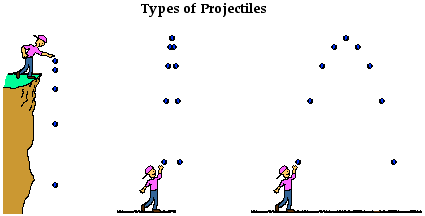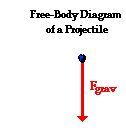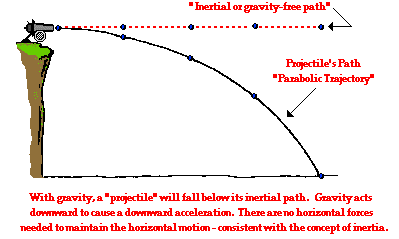Vectors - Motion and Forces in Two Dimensions - Lesson 2 - Projectile Motion

# What is a Projectile?

In Unit 1 of the Physics Classroom Tutorial, we learned a variety of means to describe the 1-dimensional motion of objects. In Unit 2 of the Physics Classroom Tutorial, we learned how Newton's laws help to explain the motion (and specifically, the changes in the state of motion) of objects that are either at rest or moving in 1-dimension. Now in this unit we will apply both kinematic principles and Newton's laws of motion to understand and explain the motion of objects moving in two dimensions. The most common example of an object that is moving in two dimensions is a projectile. Thus, Lesson 2 of this unit is devoted to understanding the motion of projectiles.

###Defining Projectiles

A projectile is an object upon which the only force acting is gravity. There are a variety of examples of projectiles. An object dropped from rest is a projectile (provided that the influence of air resistance is negligible). An object that is thrown vertically upward is also a projectile (provided that the influence of air resistance is negligible). And an object which is thrown upward at an angle to the horizontal is also a projectile (provided that the influence of air resistance is negligible). A projectile is any object that once projected or dropped continues in motion by its own inertia and is influenced only by the downward force of gravity.By definition, a projectile has a single force that acts upon it - the force of gravity. If there were any other force acting upon an object, then that object would not be a projectile. Thus, the free-body diagram of a projectile would show a single force acting downwards and labeled force of gravity (or simply Fgrav). Regardless of whether a projectile is moving downwards, upwards, upwards and rightwards, or downwards and leftwards, the free-body diagram of the projectile is still as depicted in the diagram at the right. By definition, a projectile is any object upon which the only force is gravity.

### Projectile Motion and InertiaMany students have difficulty with the concept that the only force acting upon an upward moving projectile is gravity. Their conception of motion prompts them to think that if an object is moving upward, then there must be an upward force. And if an object is moving upward and rightward, there must be both an upward and rightward force. Their belief is that forces cause motion; and if there is an upward motion then there must be an upward force. They reason, "How in the world can an object be moving upward if the only force acting upon it is gravity?" Such students do not believe in Newtonian physics (or at least do not believe strongly in Newtonian physics). Newton's laws suggest that forces are only required to cause an acceleration (not a motion). Recall from the Unit 2 that Newton's laws stood in direct opposition to the common misconception that a force is required to keep an object in motion. This idea is simply not true! A force is not required to keep an object in motion. A force is only required to maintain an acceleration. And in the case of a projectile that is moving upward, there is a downward force and a downward acceleration. That is, the object is moving upward and slowing down.

To further ponder this concept of the downward force and a downward acceleration for a projectile, consider a cannonball shot horizontally from a very high cliff at a high speed. And suppose for a moment that the gravity switch could be turned off such that the cannonball would travel in the absence of gravity? What would the motion of such a cannonball be like? How could its motion be described? According to Newton's first law of motion, such a cannonball would continue in motion in a straight line at constant speed. If not acted upon by an unbalanced force, "an object in motion will ...". This is Newton's law of inertia.Now suppose that the gravity switch is turned on and that the cannonball is projected horizontally from the top of the same cliff. What effect will gravity have upon the motion of the cannonball? Will gravity affect the cannonball's horizontal motion? Will the cannonball travel a greater (or shorter) horizontal distance due to the influence of gravity? The answer to both of these questions is "No!" Gravity will act downwards upon the cannonball to affect its vertical motion. Gravity causes a vertical acceleration. The ball will drop vertically below its otherwise straight-line, inertial path. Gravity is the downward force upon a projectile that influences its vertical motion and causes the parabolic trajectory that is characteristic of projectiles.A projectile is an object upon which the only force is gravity. Gravity acts to influence the vertical motion of the projectile, thus causing a vertical acceleration. The horizontal motion of the projectile is the result of the tendency of any object in motion to remain in motion at constant velocity. Due to the absence of horizontal forces, a projectile remains in motion with a constant horizontal velocity. Horizontal forces are not required to keep a projectile moving horizontally. The only force acting upon a projectile is gravity!

### We Would Like to Suggest ...Sometimes it isn't enough to just read about it. You have to interact with it! And that's exactly what you do when you use one of The Physics Classroom's Interactives. We would like to suggest that you combine the reading of this page with the use of our Projectile Motion Simulator. You can find it in the Physics Interactives section of our website. The simulator allows one to explore projectile motion concepts in an interactive manner. Change a height, change an angle, change a speed, and launch the projectile.

Next Section: